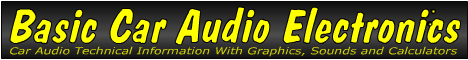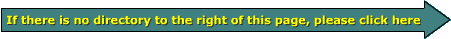Email   Home Pagex

 Web www.bcae1.com www.bcot1.com
Use F11 to go to full screen
viewing if using Google
Translate.

 Reactance When capacitors and inductors (coils) are connected to an AC voltage source, they oppose the flow of electrical current. The amount of opposition depends on the value of the component (capacitor or inductor) and the applied frequency. Capacitors block direct current (DC) and tend to block lower frequencies. This is why they're useful in high-pass filters/crossovers. Inductors pass low frequencies with little or no opposition and tend to block higher frequencies. This is why they are used to block mids and highs when connected in series with a low frequency speaker. In theory a perfect inductor or capacitor has no resistance. The reactance of a capacitor or inductor is similar to the resistance of a resistor. The biggest difference is that a resistor essentially opposes AC equally at all frequencies. The reactance of a coil (inductor) or capacitor varies with frequency.

 Use this program to calculate the reactance for inductors and capacitors at various frequencies. You should notice that the reactance increases for inductors as the frequency increases. The reactance for the capacitor decreases as the frequency increases.

 Data Input: Capacitor Value? microfarads Inductor Value? millihenries Test Frequency? hertz Data Output: Capacitive Reactance = ohms Inductive Reactance = ohms

Capacitive Reactance =
 12*3.14*applied frequency*capacitor value

 Inductive Reactance = 2*3.14*applied frequency*inductor value

Click HERE to visit a friend's new car audio tech site.

```

```